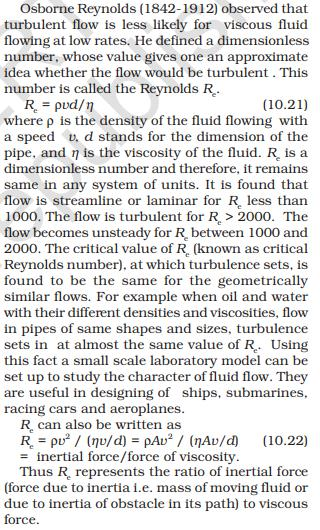Courses

# Test: Non-Ideal Fluids

## 10 Questions MCQ Test Physics Class 11 | Test: Non-Ideal Fluids

Description
This mock test of Test: Non-Ideal Fluids for JEE helps you for every JEE entrance exam. This contains 10 Multiple Choice Questions for JEE Test: Non-Ideal Fluids (mcq) to study with solutions a complete question bank. The solved questions answers in this Test: Non-Ideal Fluids quiz give you a good mix of easy questions and tough questions. JEE students definitely take this Test: Non-Ideal Fluids exercise for a better result in the exam. You can find other Test: Non-Ideal Fluids extra questions, long questions & short questions for JEE on EduRev as well by searching above.
QUESTION: 1

### The viscosity of liquids _________ with increase in temperature.

Solution:

The viscosity of liquids decreases with increase in temperature. The cohesive forces between molecules of liquids decrease as temperature increases. At high temperature the molecules of liquids have high energy and overcome strong cohesive forces and move freely. Hence, viscosity of liquids decreases with increase in termperature.

QUESTION: 2

Solution:
QUESTION: 3

### As the velocity of a body increases, the viscous force on it

Solution:

Drag acts as an opposing force to acceleration. Drag will never increase velocity, but whilst the forces acting to move the object are greater than the drag or resistive forces then the object will continue to accelerate, thus increasing velocity. Simplified, drag is caused by the object colliding with air particles. As the object moves faster, the object will come into contact with more particles and this will increase the effect of drag. Drag decreases acceleration and therefore velocity, but an increase in velocity will increase drag forces.

QUESTION: 4

A value of Reynold’s number _________ indicates turbulence.

Solution:QUESTION: 5

The viscosity of gases ________ with increase in temperature.

Solution:

According to kinetic theory of gases viscosity in gases increases with increase in temperature because increase in kinetic energy will increase the frequency of collision between the molecules.

QUESTION: 6

Terminal velocity of a spherical body of density ρ and radius r moving through a liquid of density ρ’ is given by

Solution:
QUESTION: 7

The relative velocity between two parallel layers of water is 10 cm/s and the perpendicular distance between them is 0.1 cm. The velocity gradient is

Solution:

Velocity gradient = dv/dx
dv = 10 cm/s
dx = 0.1 cm
dv/dx = 10/0.1 = 100cm/s cm

QUESTION: 8

Force of viscosity acting on a spherical body of radius r moving with velocity v through a fluid of viscosity η is given by

Solution:

Stoke’s Law calculates the drag force on the sphere as it travels through a fluid.
F = 6 πrηv

QUESTION: 9

The quantity which decides the nature of flow of a viscous liquid through a pipe is known as

Solution:

The Reynolds Number (Re) is proportional to the ratio between the momentum of a flowing fluid and its viscosity. The lower Re, the more viscosity governs the flow behavior, so the more it sticks to a surface, and the flow is laminar. The higher the Re, the more its velocity governs, so the less it sticks to surface and the flow is turbulent. Laminar flow is straight lines. Turbulent flow is eddies and swirling vortexes.

QUESTION: 10

Range of Reynold’s number for the laminar flow of a liquid is

Solution: